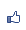[Excel VBA] ColorIndex 之 57 色演色表 (實際展示)
2018/06/16 22:17:26瀏覽11589｜回應1｜推薦1

*註：參閱 https://blog.udn.com/WayCheng/2705003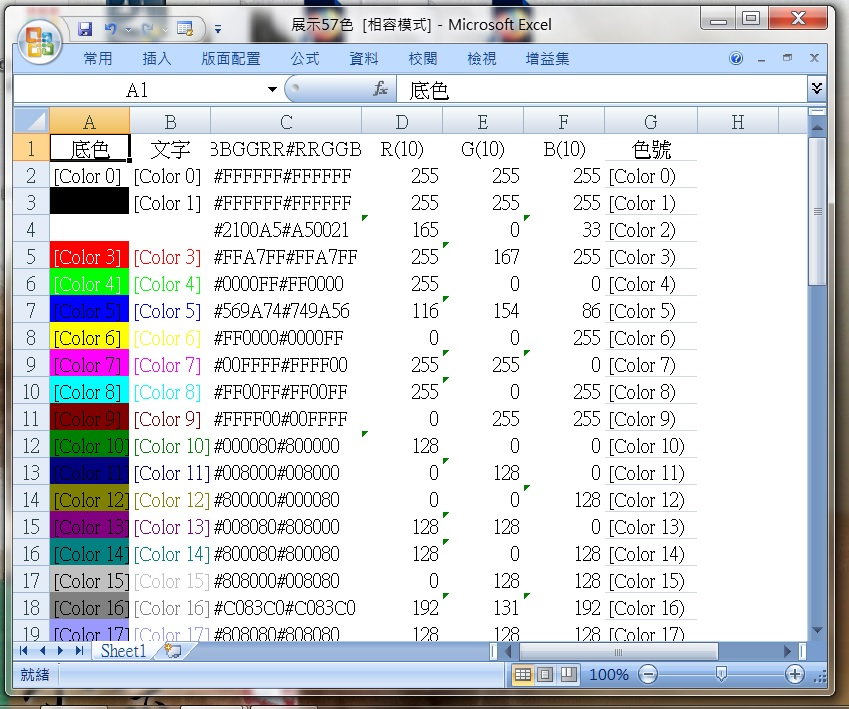A 欄：呈現該色彩用於儲存格底色 (interior)

B 欄：呈現該色彩用於儲存格裡的文字 (font)

C 欄：以十六進位數字表示該顏色的三原色值 (依 BBGGRR 和 RRGGBB 兩種順序並列)

D、E、F 欄：以十進位數字分別表示該顏色的 R、G、B 值

G 欄：該列的色號 (ColorIndex)

Sub Demo57Colors()

Coder: Way Cheng

Application.ScreenUpdating = False            暫時停止螢幕更新

Application.Calculation = xlCalculationManual 暫時停止自訂公式的執行

Dim i As Long

Dim strBGR As String, strRGB As String

Cells(1, 1) = "底色"

Cells(1, 2) = "文字"

Cells(1, 3) = "#BBGGRR#RRGGBB"

Cells(1, 4) = "R(10)"

Cells(1, 5) = "G(10)"

Cells(1, 6) = "B(10)"

Cells(1, 7) = "色號"

For i = 0 To 56

Cells(i + 2, 1).Interior.ColorIndex = i      A欄：背景底色設為 i 號色彩

Cells(i + 2, 1).Value = "[Color " & i & "]"  A欄: 填入文字 (色號)

Cells(i + 2, 2).Font.ColorIndex = i          B欄: 文字色彩設為 i 號色彩

Cells(i + 2, 2).Value = "[Color " & i & "]"  A欄: 填入文字 (色號)

strBGR = Right("000000" & Hex(Cells(i + 2, 1).Interior.Color), 6)  取A欄底色值 6 nibbles為 "BBGGRR"

strRGB = Right(strBGR, 2) & Mid(strBGR, 3, 2) & Left(strBGR, 2)    然後轉成 "RRGGBB" (strRGB)

Cells(i + 2, 3) = "#" & strBGR & "#" & strRGB & ""   C欄: 以16進位顯示 GBR 與 RGB

Cells(i + 2, 4).Formula = "=Hex2dec(""" & Right(strBGR, 2) & """)"  D欄: Red 值以十進位顯示

Cells(i + 2, 5).Formula = "=Hex2dec(""" & Mid(strBGR, 3, 2) & """)" E欄: Green 值以十進位顯示

Cells(i + 2, 6).Formula = "=Hex2dec(""" & Left(strBGR, 2) & """)"   F欄: Blue 值以十進位顯示

Cells(i + 2, 7) = "[色彩 " & i & "]"     G欄: 儲存格自訂格式時可以這樣寫

Next i

done:

Application.Calculation = xlCalculationAutomatic  公式恢復執行

Application.ScreenUpdating = True                 螢幕恢復更新

End Sub

※2019/8/21 修訂程式碼，感謝吳國棟君不吝指正。

( 知識學習其他 )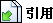引用網址：https://classic-blog.udn.com/article/trackback.jsp?uid=WayCheng&aid=112537087回應文章 選擇排序方式 　最新發表 　最舊發表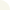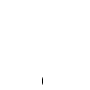ThisIsTheWay 等級：6 留言｜加入好友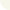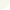2019/08/21 18:25 吳國棟先生，謝謝您! 我修改了！